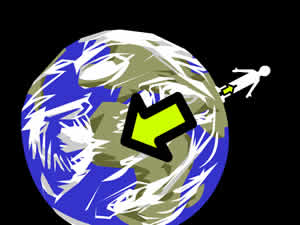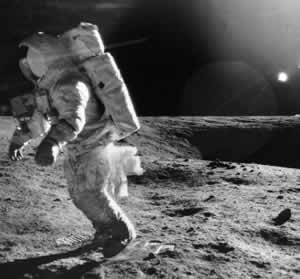# Difference between Earth and Moon Gravity

##### Key Difference: The primary difference between the gravities of the Earth and the Moon is that the moon’s gravity is weaker due to the moon being smaller in size, whereas, the earth is bigger in size, and has more massive objects that exert more gravity.

To be aware of the terms Earth's gravity and Moon's gravity, let’s understand what is Gravity? Gravity is a force which binds together all matter (which is anything a person can physically touch). In simple terms, more the matter, more is the gravity, so things that have a lot of matter such as planets, moons, and stars, pull more strongly.Each and every person is falling towards the Earth at an accelerating rate of 9.8 meters/second². Gravity basically comes from the mass. The amount of gravity reduces gradually with the reduction in distance. As a result, the force of gravity that one feels on the surface of Earth is much different from what one would experience at the distance of the Moon, or at a distance further from it. The force of gravity of Earth also changes depending on where a person is standing on it. The primary reason for this is because the Earth is rotating. The gravity of Earth at the equator is 9.789 m/s2, while the force of gravity at the poles is 9.832 m/s2. In other words, one weighs more at the poles than one does at the equator because of this central force.

Also see: The difference between the universe and the solar systemGravity on the Moon is much less. The moon is smaller and has much less mass, it pulls with less gravity. Actually, if one could stand on the plane of the Moon, he would experience only 17%  force of gravity that one would experience on Earth. With gravity on the moon so low, a person can even jump higher than he/she can on Earth. The acceleration due to gravity on the surface of the Moon is 1.62 m/s2, about 16.6% of that on the Earth's surface.

## Difference between Earth Gravity and Moon Gravity:

 Earth Gravity Moon Gravity Gravity Gravity on the surface of the earth is equal to 9.8 m/s2. The gravity of the moon on the lunar surface is only 1.63 m/s2. Mapped Very precisely mapped Poorly mapped Atmosphere Strong enough to sustain an atmosphere. There is no atmosphere available. Size Earth is bigger than the moon Moon is smaller than the earth.

Also see the difference between a galaxy and the solar system

Image Courtesy: brainpop.com, earthsky.org